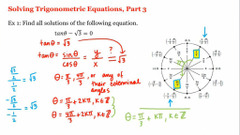## Pre-Calculus

 所在平台: Coursera 课程类别: 数学Explore 1600+ online courses from top universities. Join Coursera today to learn data science, programming, business strategy, and more.#### 课程详情

This course is taught so that students will acquire a solid foundation in algebra and trigonometry. The course concentrates on the various functions that are important to the study of the calculus. Emphasis is placed on understanding the properties of linear, piece-wise, exponential, logarithmic and trigonometric functions. Students will learn to work with various types of functions in symbolic, graphical, numerical and verbal form.

#### 课程大纲

This course will be taught over ten weeks, with materials released on a weekly basis. Below you will find the weekly topic schedule.

Week One: Algebra Review. Linear Equations. Linear Inequalities. Absolute Value in Equations and Inequalities.
Week Three: Graphing Functions. Transformations of Functions. Quadratic Functions.
Week Four: Inverse Functions. Exponential Functions. Logarithmic Functions.
Week Five: Exponential and Logarithmic Equations. Review. Midterm Exam.
Week Six: Angles and their Measure. Trigonometric Functions: A Unit Circle. Solving Right Triangles.
Week Seven: Properties of Trigonometric Functions. Inverse Trigonometric Functions.
Week Eight: Basic Identities and Their Use. Sum, Difference and Cofunction, Double-Angle and Half-Angle Identities.
Week Nine: Trigonometric Equations. Law of Sines. Law of Cosines
Week Ten:  Review and Final Exam.

#### 课程评论(0条)#### 课程简介

This class prepares students for eligibility to enroll in the first semester of single variable calculus.

#### 6人关注该课程